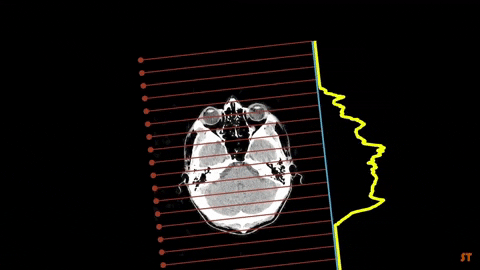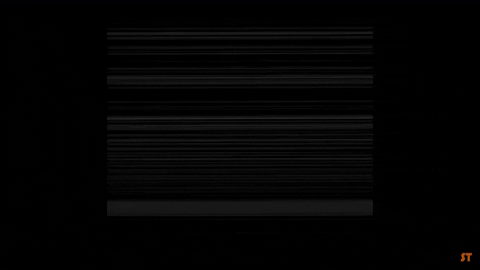# Binary Tomography

Tomography (from greek tomos = slice or section and grapho= to write or to describe) is an image reconstruction method by using sections generated from any kind of penetrating wave passing through a not accessible or not visible object..Binary image is an image whose pixels have only two possible intensity values: 0 (black) and 1 (white). It is useful because of an easy separation of an object from the background.Binary Tomography (BT) deals with the reconstruction of binary images by using a limited number of their projections. It is a special case of Discrete Tomography (DT), an image reconstruction technique dealing with a finite discrete set of functions. With DT, reconstruction of an image is possible with a much smaller dataset than for the Computerized Tomography (CT).

Binary Tomography reconstruction methods require data from at least two or even more projection directions. However, the problem with binary image reconstruction is that several binary images can have the same projections especially when only one or two projections are given, which makes the problem undetermined. This is why some additional a priori information is needed in the reconstruction process, for example geometrical or material descriptors of the observed image or energy functions.

The basic BT reconstruction problem can be represented by a set of linear equations written in matrix form as

A u = b, A ∈ RM×N, u ∈ {0, 1}N, b ∈ RM

where:

• A is the projection matrix whose each row represents one projection ray,

• b is the projection vector containing the projected values,

• u is the vector of the unknown binary image to be reconstructed.

The reconstruction problem deals with finding the solution vector u from the linear system of equations, where the projection matrix and the projection vector are given. As the system is in most cases underdetermined (N > M), some additional regularization is needed to achieve satisfactory reconstruction of the original images.

## Applications

Non-destructive material testingTillage-induced effects on SOC through changes in aggregate stability and soil pore structure

Soil slice image from X-ray computer tomography of CT (a) and NT (b); Soil binary image after segmentation of CT (c) and NT (d); Soil 3D image of CT (e) and NT (f). In a) and b), dark areas are pores, while in c) and d), white areas represent pores and black areas represent solid material. In e) and f) white areas are the pores. The layers of the individual images are evident as 'contours' on the white pores.Gas flow in near surface comet like porous structures

Binary X-Ray Micro-CT image cross section of porous medium samples used with a resolution of 5 μm. White colour is porous material and black colour is void area.A novel feature-based tracking approach to the detection, localization, and 3-D reconstruction of internal defects in hardwood logs using computer tomography

An example CT image and the result of knot detection using (1): a original CT image, and b binary image.The goal is to reconstruct a given binary images dataset by using projection techniques and improve the reconstructed images quality by using optimization and regularization techniques. We employed four methods to reconstruct the given binary images and compared the results of the applied methods in order to compare the performance of each one.## Methodology

### The approach chosen for the solution of the project task consists of image reconstruction by using projection from several different angles and optimization of the acquired reconstruction by using genetic algorithms.

The approach chosen for the solution of the project task consists of :

•#### Dataset preparation

Number of projections are defined by setting up a function for the projection angles. Matrices A and b are calculated from the number of rays and number of projections. for different reconstruction methods used different image resolutions were used.

•#### Image reconstruction by basic back projection

the images were reconstructed by using iRadon function from Matlab with filter set to none.

•#### Image reconstruction by filtered back projection

The images were reconstructed by using iRadon function from Matlab with arguments with filter set to Ram-Lak filter.

•#### Image reconstruction by genetic algorithm optimization

The images were reconstructed by using genetic algorithm function with initial population set to output of filtered back projection.

•#### Image reconstruction by gradient descent optimization

The images were reconstructed by using gradient descent method with implemented gradient.

## Results## Team#### Martin

Data Analysis and Programming#### Rozemarijn

Data Analysis and Programming#### Iva

Research and Website Design#### Ivana

Research and Report Writing